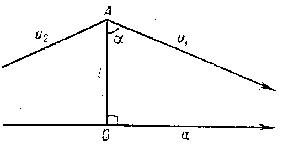# Lobachevskii function

The angle of parallelism in Lobachevskii geometry is a function that expresses the angle $\alpha$ between the line $u _ {1}$( or $u _ {2}$) (see Fig.) and the segment $OA$ perpendicular to a line $a$ parallel to $u _ {1}$( or $u _ {2}$) in terms of the length $l$ of the segment $OA$:

$$\alpha = \Pi ( l) = 2 { \mathop{\rm arc} \mathop{\rm tan} } e ^ {- l / R } ,$$

where $R$ is a positive constant that corresponds to the scale of measurement of distances.Figure: l060020a

The Lobachevskii function is a continuous monotone decreasing function with values between $\pi /2$ and 0:

$$\lim\limits _ {l \rightarrow 0 } \Pi ( l) = \frac \pi {2} ,\ \lim\limits _ { l \rightarrow \infty } \Pi ( l) = 0.$$

It was introduced by N.I. Lobachevskii in 1826.

## Contents

How to Cite This Entry:
Lobachevskii function. Encyclopedia of Mathematics. URL: http://encyclopediaofmath.org/index.php?title=Lobachevskii_function&oldid=47674
This article was adapted from an original article by A.B. Ivanov (originator), which appeared in Encyclopedia of Mathematics - ISBN 1402006098. See original article